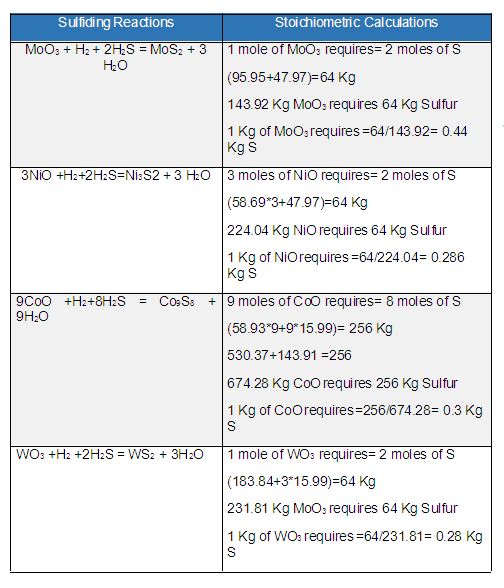# Calculating the Amount of DMDS Required to Complete the Sulfiding

Home Forums Petro Solutions Forum Hydroprocessing Calculating the Amount of DMDS Required to Complete the Sulfiding

• Author
Posts
• #1516

The required quantity of Sulfur depends upon the amount of metals present in the catalyst. The composition or amount of the catalyst metal to be sulfided is provided by the catalyst supplier. So first find the exact composition of the catalyst then calculate the stoichiometric amount of Sulfur needed for sulfiding by these reactions as given in the table below.
Details about the sulfiding procedure can be found at Sulfiding of Hydrotreating Catalysts and its Troubleshooting

Molecular weights are of Tungsten 183.84, Molybdenum 95.95, Oxygen 15.99, Hydrogen 1.0, Nickel 58.69, Sulfur 32, and Cobalt 58.93.From the stoichiometric calculations as per the above image, the summary is as follows;
1 Kg of MoO3 requires 0.44 Kg of S
1 Kg of NiO requires 0.286 Kg of S
1 Kg of CoO requires 0.3 Kg of S
1 Kg of WO3 requires 0.28 Kg of S

For example, the hydrotreating catalyst having the composition 12 % Mo (12 Ton), 5 % (5 Ton) Ni, and 5 % Co (5 Ton), then 100 Ton of catalyst will require the sulfur using the unit method as follow;

1 Ton of MoO3 requires = 0.44 Ton of S
12 Tons of MoO3 requires = 12* 0.44= 5.28 Ton Sulfur
1 Ton of NiO requires = 0.286 Ton of S
5 Ton of NiO requires = 5*0.286 = 1.43 Ton
1 Ton of CoO requires = 0.3 Ton of S
5 Ton of CoO requires = 0.3*5 = 1.5 Ton of S
Total Sulfur required for Sulfiding = 5.28+1.43+1.5= 8.21 Tons

Total Sulfur required for sulfiding the 100 Ton of catalyst having composition 12 % Mo, 5 % Ni and 5 % Co is 8.21 Tons of Sulfur.
The amount of sulfiding agent DMDS (contains 68 % weight of Sulfur) can be found as follows;
0.68 Ton of Sulfur = 1 Ton of DMDS
1 Ton of Sulfur = 1/0.68 =1.47 Tons of DMDS
8.21 Ton of Sulfur = 8.21*1.47= 12 Tons of DMDS

So for Sulfiding 100 ton Hydrotreating catalyst with the composition stated above, the stoichiometric amount required is 12 tons of DMDS. But the actual amount required to ensure complete sulfiding would be more than 12 tons (15~17 Tons).